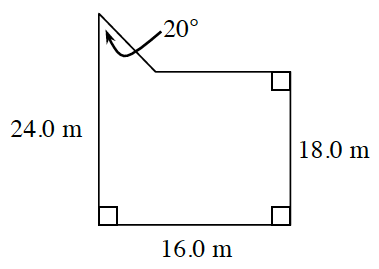### Home > INT2 > Chapter 4 > Lesson 4.1.5 > Problem4-52

4-52.

Calculate the area and the perimeter of the figure below. Be sure to organize your work so you can explain your method later.Can you break the figure into familiar shapes?

Subtract $18.0$ m from $24.0$ m to calculate the height of the triangle.

Use trigonometry to calculate the length of the second leg of the triangle.

$\text{area}\approx294.6$ sq m